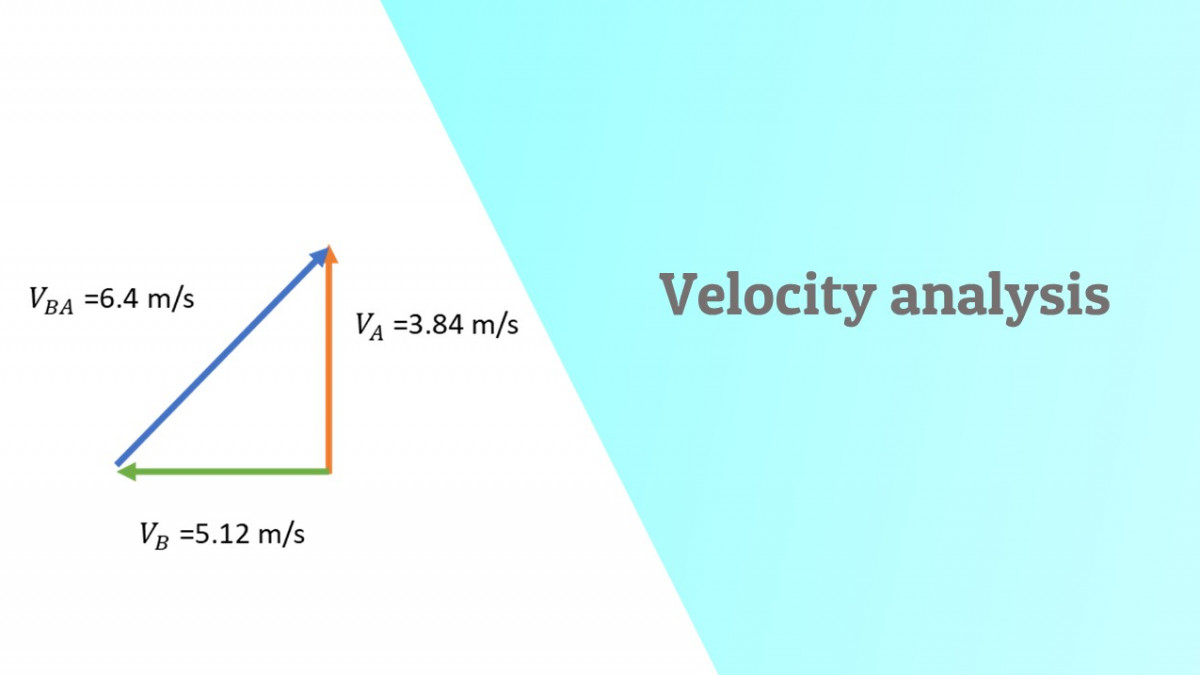# Velocity analysis of a 4 bar link mechanismA brief breakdown on how to perform a graphical velocity vector analysis on a 4 bar link mechanism

Velocity of various points on a mechanism can be analyzed analytically or graphically using vectors

Analyzing a mechanism graphically means defining the magnitude and direction of the velocity vectors.

Here is a walkthrough on how we can derive the Velocity diagram when solving graphically.

Consider a rigid body AB with two different velocities at two points A and B

The velocity difference vector will give the relative velocity of point B with respect to point A.

Velocity analysis of coupler of 4 bar link mechanism

Since links OA and BD are both rotating about fixed centers they will have linear velocities respectively at the instant as shown

How to find absolute velocity of point C?

Same way we found velocity difference for B with respect to A we can do the same for C with respect to A

Also we can find velocity difference for C with respect to B too

Creating the Velocity polygon and bringing all the vectors into one diagram

Categories: Mechanism and Machine design